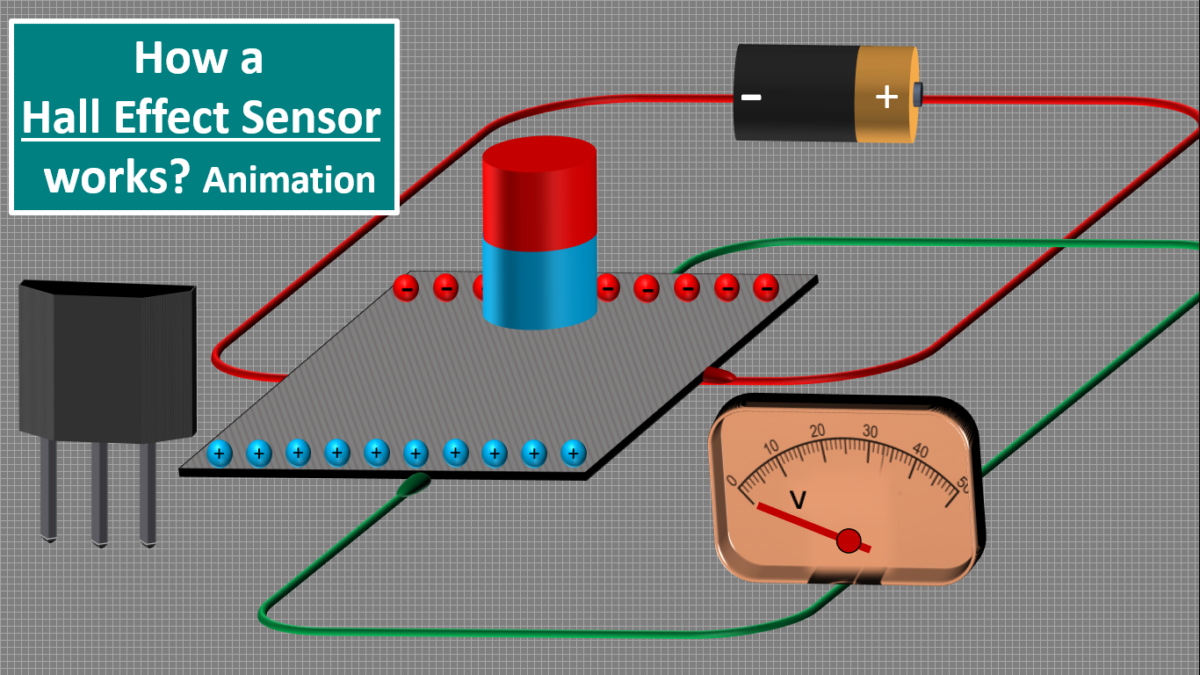# What is HallEffect sensor.Working of Hall Effect Sensor.Hall Effect Proximity Sensor Working.Hall sensor, Hall Element.Hall effect sensor or hall sensor is a magnetic sensor which generates electrical signal proportional to the magnetic field applied on it.

The Electrical signals generated by the hall effect sensors are then further processed by a user-specific electronic circuit to give the desired output.

Hall effect sensors are non-contact sensors which means they do not have to come in contact with a physical element.

They can produce either digital or analog output signal depending on their design and indented function.

Hall Effect sensors are widely used in industrial applications like current sensing, position detection and contactless switching.

Working principle of Hall Effect Sensors.

Hall effect sensor works on the principle of Hall effect.

When a conductive plate is connected to a circuit with a battery, a current starts flowing from one end of the battery to the other end through the Conductive plate.

The Charge carriers will flow in a straight line from one end of the plate to the other end. as charge carriers are in motion they will produce a magnetic field.

Now when you place a magnet near the plate the magnetic field of the magnet will distort the magnetic field of the charge carriers. this will interrupt the straight flow of charge carriers.

The Force which interrupts the direction of flow of charge carriers is known as Lorentz Force.

Due to the distortion in the magnetic field of the charge carriers the negatively charged electrons will be deflected to one side of the plate and the positively charged holes will be deflected to the other side.

Thus a potential difference known as hall voltage will be generated between both sides of the plate which can be measured using a meter. this effect is known as hall effect.

if the magnetic field is removed the hall voltage will be zero. if the magnetic field is reversed the hall voltage will also be reversed.

Construction and Working of Hall Effect Sensor.

Hall effect sensors consist of a thin piece of rectangular p-type semiconductor material like Gallium Arsenide, Indium Arsenide, Silicon etc.

This P-type semiconductor material is called Hall Element. Continuous current is allowed to flow through this Hall Element.

Hall Element has output connections perpendicular to the direction of current flow. when the device is placed within a magnetic field the magnetic flux lines exert a force on the semiconductor material which deflects the electrons and holes which are the charge carriers to either side of the Hall Element.

As these electrons and holes move sideward a potential difference is produced between the two sides of the semiconductor material. The output voltage is proportional to the magnetic field strength.

The Output Voltage is very small and requires additional electronics to achieve useful voltage levels. when the whole element is combined with the associated electronics it forms a Hall Effect Sensor.

Types of Hall Effect Sensors:
Hall effect sensors can be classified into two types on the basis of output.

1. Analog Output Hall Effect sensor

2. Digital Output Hall Effect Sensor.

Analog output hall effect sensor
The Analog Output hall effect sensor contains a voltage regulator a hall element and an amplifier. This type of sensor gives analog output proportional to the magnetic field strength.

If the hall voltage is measured when no magnetic field is present the output voltage will be zero but if voltage at each output terminal of the whole element is measured with respect to ground a non-zero voltage will appear and this voltage will be the same at each output terminal. This voltage is known as Common mode voltage.

In order to nullify this common mode voltage a Differential Amplifier is used so as to amplify only the potential difference that is the hall voltage and nullify the common mod voltage.

The Hall voltage is a function of input current the purpose of the regulator in the circuit is to hold this current constant so that the output of the sensor only reflects the intensity of the magnetic field.

Digital Output Hall Effect Sensor.

The Basic analog output device which we had discussed earlier can be converted into a digital output sensor with the addition of a Schmitt Trigger Circuit.

The Schmitt trigger compares the output of the differential amplifier with a preset value. when the amplifier output exceeds the preset value the Schmitt trigger turns ON.

Likewise, when the amplifier output falls below the preset value the Schmitt trigger turns OFF. thus this sensor has an output that is just one of two states ON or OFF.

Do u want to know anything more about Instrumentation?